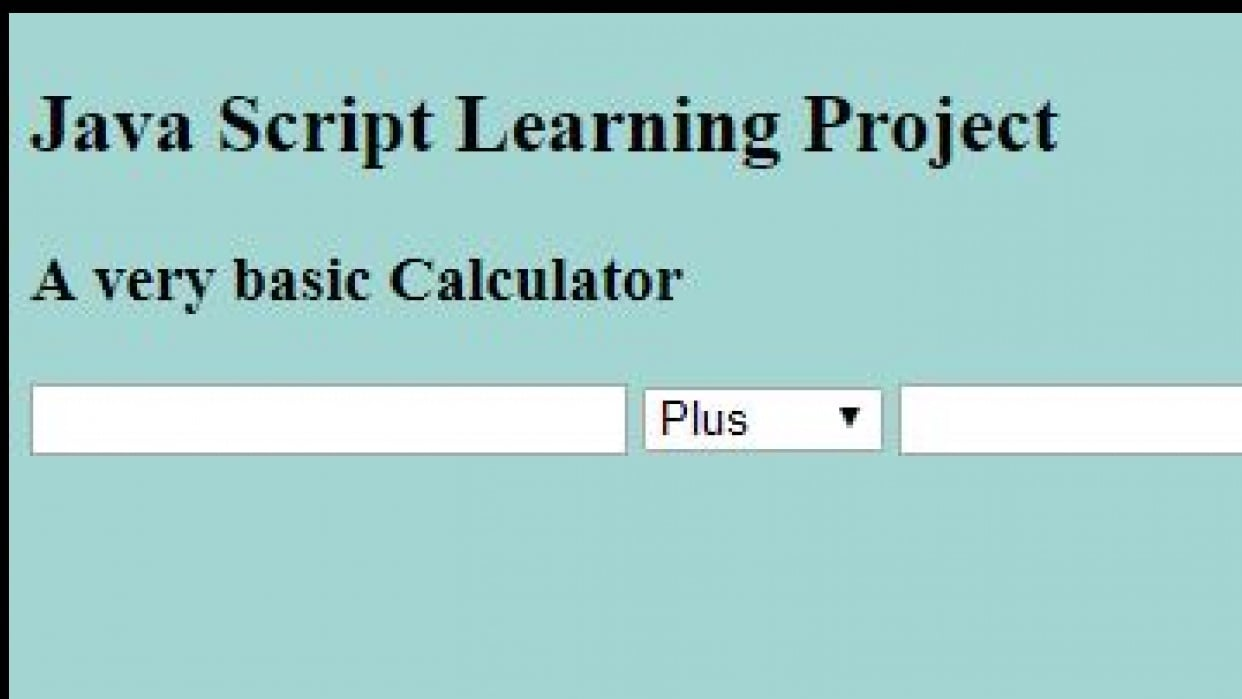## A Very Basic CalculatorHTML:

<!DOCTYPE html>
<html>
<title>Calculator</title>
<body>
<section>
<h1>Java Script Learning Project</h1>
<h3>A very basic Calculator</h3>
</section>
<section>
<form>
<input type="number" name="numberOne" id="num1" />
<select id="selection">
<option value="minus">Minus</option>
<option value="multiply">Multiply</option>
<option value="divide">Divide</option>
</select>
<input type="number" name="numberTwo" id="num2">
<button type="button" onclick="calculateFN();">Calculate</button>
<div id="result">
</div>
</form>
</section>

<script type="text/javascript" src="calculator.js"></script>
</body>
</html>

JavaScript:

function calculateFN() {

//a variable to target the ID of HTML element where the result of calculation is displayed
var result = document.querySelector("#result");

//variables for the numbers which user is inputing, needed to access value and be in number format.
var numberOne = Number(document.querySelector("#num1").value);
var numberTwo = Number(document.querySelector("#num2").value);

//separate variable for each calculation (best, shorter option would be to include them into //results, but that is how i originally solved it)
var plus = numberOne + numberTwo;
var minus = numberOne - numberTwo;
var divide = numberOne / numberTwo;
var multiply = numberOne * numberTwo;

//variable to target "select" element (the only select element on page, otherwise i would use ID)
var selectElement = document.querySelector("select")

//variable to target the value of option of the select element (couldn't solve it, had to google and research it)
var select = selectElement.options[selectElement.selectedIndex].value;

//a variable for storing the result
var calculatedResult;

//results
calculatedResult = plus;
} else if (select == "minus") {
calculatedResult = minus;
} else if (select == "divide") {
calculatedResult = divide;
} else if (select == "multiply") {
calculatedResult = multiply;
}
//to show results in targeted HTML
result.innerHTML = calculatedResult;
}

/*
Learning Process:
Originally used document.querySelector("#num1"); - returned NaN error (Not a Number - info we got from previous lessons)
Changed to: document.querySelector("#num1").value; - got a tip to use value, encountered a problem when numbers won't add up but just joined together (5+7 = 57 : 5 and 7 joined together)
Changed to Number(document.querySelector("#num1").value;) as shown in the solution.

Originally used "return" also, after looking at the solution changed it to a variable.
Had to correct a lot of syntax mistakes also, forgotten "", extra dots etc, in console.

Everything works correctly now! Tears of joy!

Thank you so much for this task, it was a very good practice, enlightening for a beginner.
I really love your courses, you explain everything super well!

*/